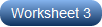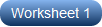# Binary and Octal Conversion Worksheets

Rich with scads of practice, our printable binary and octal conversion worksheets should be an obvious choice, if your high school students are curious to learn the different number systems. With 8 as its base, and using the numbers from 0 to 7, the octal number system has 8 unique representations and learning these is essential. Converting numbers from base-2 to base-8, writing the binary equivalent of octal numbers, and converting between octal and binary are the exercises included in these pdfs. Kick-start your practice with our free binary and octal conversion worksheet.

Converting from Binary to Octal

Binary numbers need to be converted to decimals and then to octal. Alternatively, break down each binary number in these pdfs into groups of three starting from the right. Replace each group of binary numbers with its octal equivalent to convert it.Converting from Octal to Binary

Memorize the equivalent binary values of the numbers 0 to 7, where 0 = 0, 1 = 01, 2 = 010, 3 = 011, 4 = 100, 5 = 101, 6 = 110, and 7 = 111. High school students write the binary value for each digit in the octal number to promptly switch it to base-2.Converting between Binary and Octal | MCQ

Cautiously practice the algorithmic process of conversion to base-8 from base-2 and vice versa with our printable converting between binary and octal worksheets. Students choose the binary or octal equivalent of each number as directed.